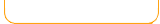Mixed Operations - Add, Subtract, Multiply, and DivideFruit Shoot - Mixed Operations Math Man - Mixed Operations Choose the number that solves the problem. Solve math problems in this arcade style game. Math Man - Expert Help the monkey collect bananas by crashing into the correct answers!Solve harder math problems in this arcade style game. Bubble Fun Math Basics Bubble Fun Math PracticePut bubbles around the sea creatures that solves the problem. (Number ranges)Put bubbles around the sea creatures that solves the problem. (Practice individual numbers) Matching Arithmetic Match the equations game. Pick the numbers that complete the equation. Fast Calculate Mahjong Mixed How fast can you calculate? Play mahjong and learn math. Quick Math Bubble Fun - Word Problems (Mixed operations) How quick are you at math?Practice decoding word problems the fun way!